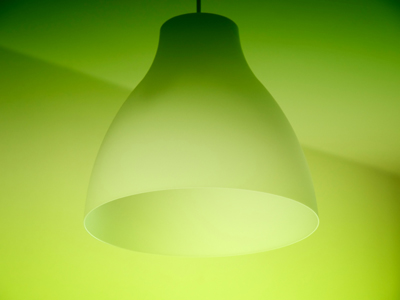A light bulb changes electrical energy into light energy.

# Electrical Circuits 01

## Test yourself on the mysteries of voltage and amps. What is a parallel circuit?

### Scroll down to play the quiz

This KS3 Science quiz challenges you on electrical circuits. An electrical circuit consists of wires, components (like switches and bulbs) and a cell or other source of power. A circuit needs to be complete for electricity to flow through it. If there is a break in the circuit, the electricity cannot pass. The amount of electricity passing through a circuit can be measured in volts and amps. The voltage tells you how much electrical energy there is. The current (number of amps) tells you how much electricity is flowing through the circuit. The amount of current flowing depends on the voltage and the resistance of the components.

There are two types of electrical circuit - series and parallel. In a series circuit, the components are arranged one after the other. If the components in a series circuit are bulbs, the more you add, the dimmer they will be. In a parallel circuit, the bulbs are connected side by side. Each one gets the same amount of energy from the battery and will all be the same brightness. If you add more bulbs in parallel, they will all be as bright as each other.

1.
What does an electric circuit need to provide energy?
A bulb
A cell
A switch
A wire
A battery contains two or more cells
2.
What is used to measure the flow of current in a circuit?
Ammeter
Battery
Rheostat
Voltmeter
Most school ammeters are now digital which makes them easier and faster to read
3.
Three bulbs connected in series:
are brighter than one bulb
are dimmer than one bulb on its own
do not light up
are the same brightness as one bulb
The energy of the cell is shared between them
4.
In order to measure the voltage across a bulb in a circuit, the voltmeter is connected in:
Not connected in the circuit
parallel with the battery
parallel with the bulb
series with the bulb
The clue is in the wording - you measure voltage across a component
5.
Which of the following does a light bulb do?
Change electrical energy into kinetic energy
Change electrical energy into light energy
Change light energy into electrical energy
Change light energy into thermal energy
Thomas Edison invented a light bulb that stayed alight. All the previous efforts had only stayed alight for a short while before the filament melted, breaking the circuit
6.
What is the unit of electric current?
Amp
Ohm
Volt
Watt
Short for ampere
7.
In order to measure the current flowing through a bulb in a circuit, the ammeter is connected in:
Not connected in the circuit
parallel with the battery
parallel with the bulb
series with the bulb
It measures the amount of electricity flowing through the circuit or component and therefore needs to be in the circuit rather than being in parallel with it
8.
If more bulbs are added in series in a series circuit:
less current flows
more current flows
no current flows
there is no change in current flow
It is the voltage from the battery or power pack that pushes the current round a circuit. As more bulbs are added to a circuit, it is harder for the battery or power pack to push the current round the circuit. This means that less current flows (so the bulbs would be dimmer than before). To make more current flow you would need to use a higher voltage. To make no current flow, you would need to break the circuit by disconnecting a wire. To keep the current the same as before, you would need to increase the voltage to compensate for the extra bulbs
9.
In a parallel circuit:
the current can flow through only one route
the current can flow through more than one route
the current does not flow
the current gets smaller
The current splits at a junction in the wires
10.
A complete circuit has:
a motor in it
a switch in it
two bulbs in it
no breaks in it
Switches are used to deliberately break a circuit so that the electricity doesn't flow round it all the time